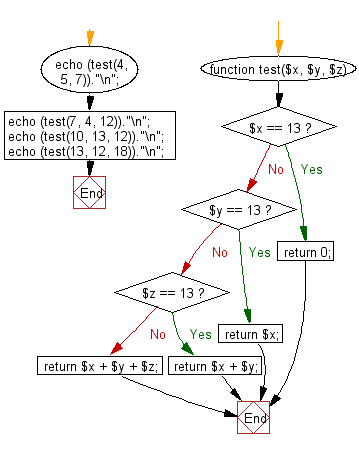﻿ PHP Exercises: Compute the sum of the three integers. If one of the values is 13 then do not count it and its right towards the sum - w3resource# PHP Exercises: Compute the sum of the three integers. If one of the values is 13 then do not count it and its right towards the sum

## PHP Basic Algorithm: Exercise-55 with Solution

Write a PHP program to compute the sum of the three integers. If one of the values is 13 then do not count it and its right towards the sum.

Sample Solution:

PHP Code :

``````<?php
function test(\$x, \$y, \$z)
{
if (\$x == 13) return 0;
if (\$y == 13) return \$x;
if (\$z == 13) return \$x + \$y;
return \$x + \$y + \$z;
}

echo (test(4, 5, 7))."\n";
echo (test(7, 4, 12))."\n";
echo (test(10, 13, 12))."\n";
echo (test(13, 12, 18))."\n";
``````

Sample Output:

```16
23
10
0
```

Flowchart:PHP Code Editor:

What is the difficulty level of this exercise?

﻿

## PHP: Tips of the Day

\$_REQUEST: This SuperGlobal Variable is used to collect data submitted by a HTML Form

Example:

This code save in a php file and run in the browser.

```<!DOCTYPE html>
<html>
<body>

<form method="post" action="<?php echo \$_SERVER['PHP_SELF'];?>">
NAME: <input type="text" name="user">
<button type="submit">SUBMIT</button>
</form>
<?php
if (\$_SERVER["REQUEST_METHOD"] == "POST") {
\$name = htmlspecialchars(\$_REQUEST['user']);
if(empty(\$name)){
echo "Name is empty";
} else {
echo \$name;
}
}
?>
</body>
</html>
```

Output:

```Owen
```